Constructing perpendicular to the line segment

Practical Geometry
Serial order wise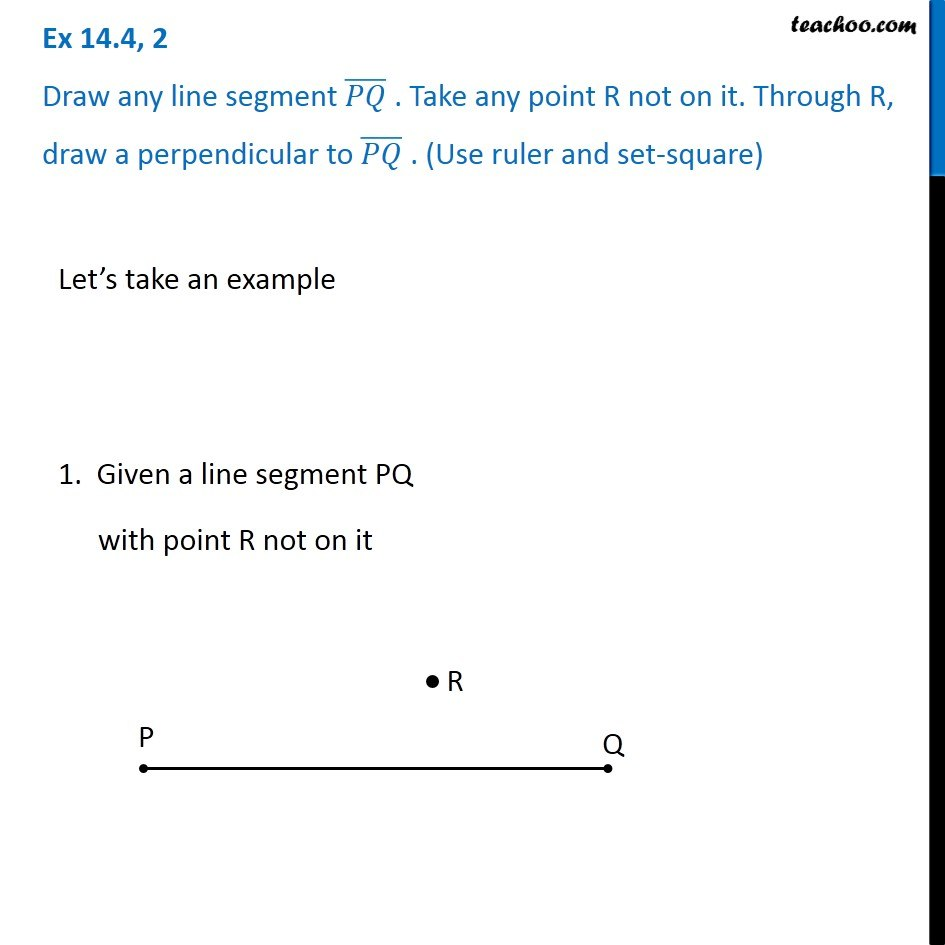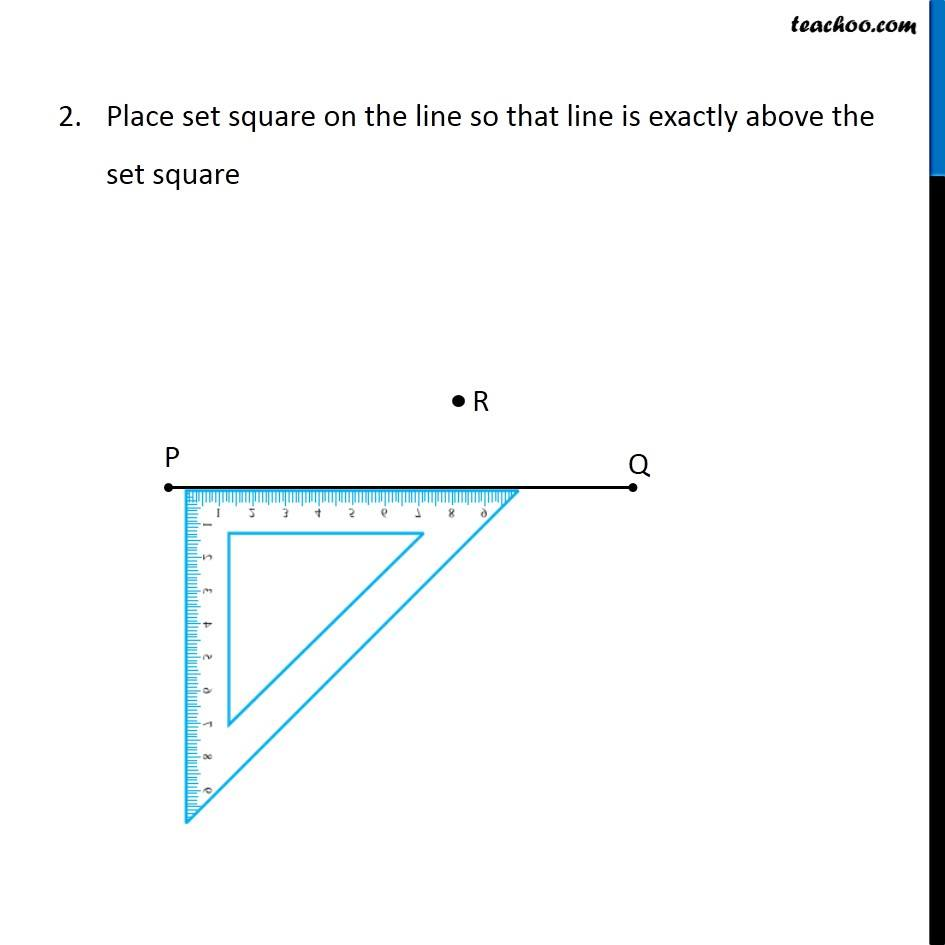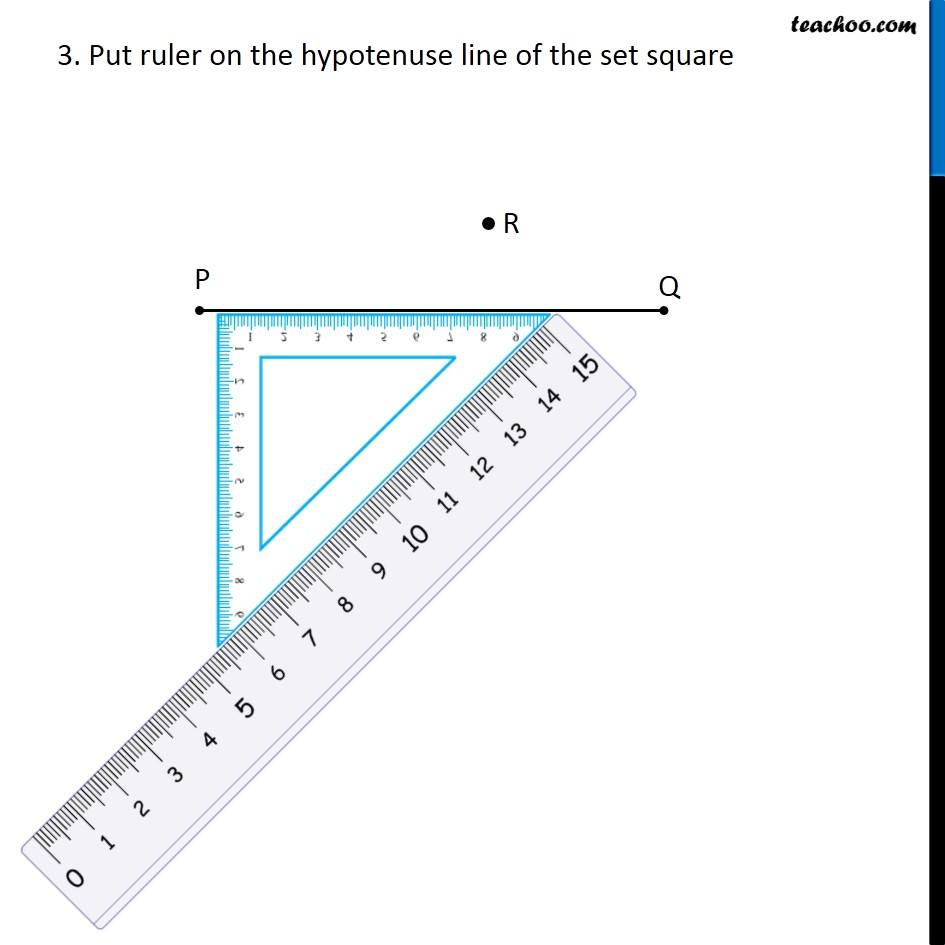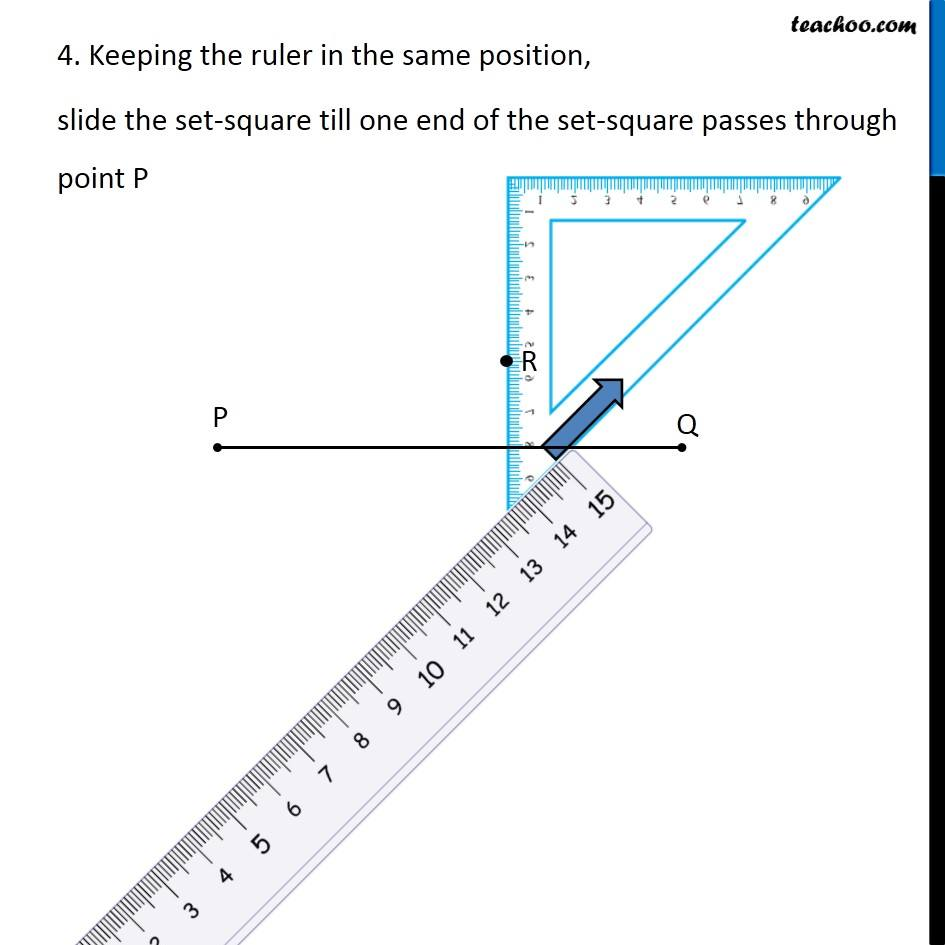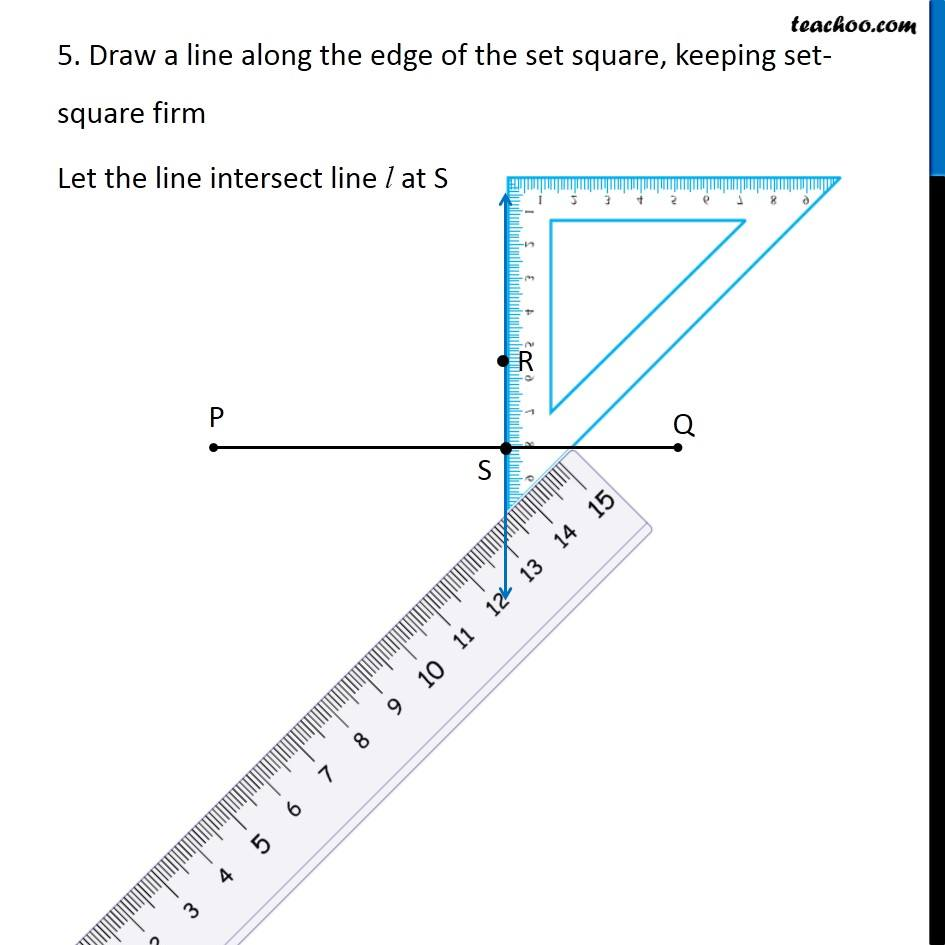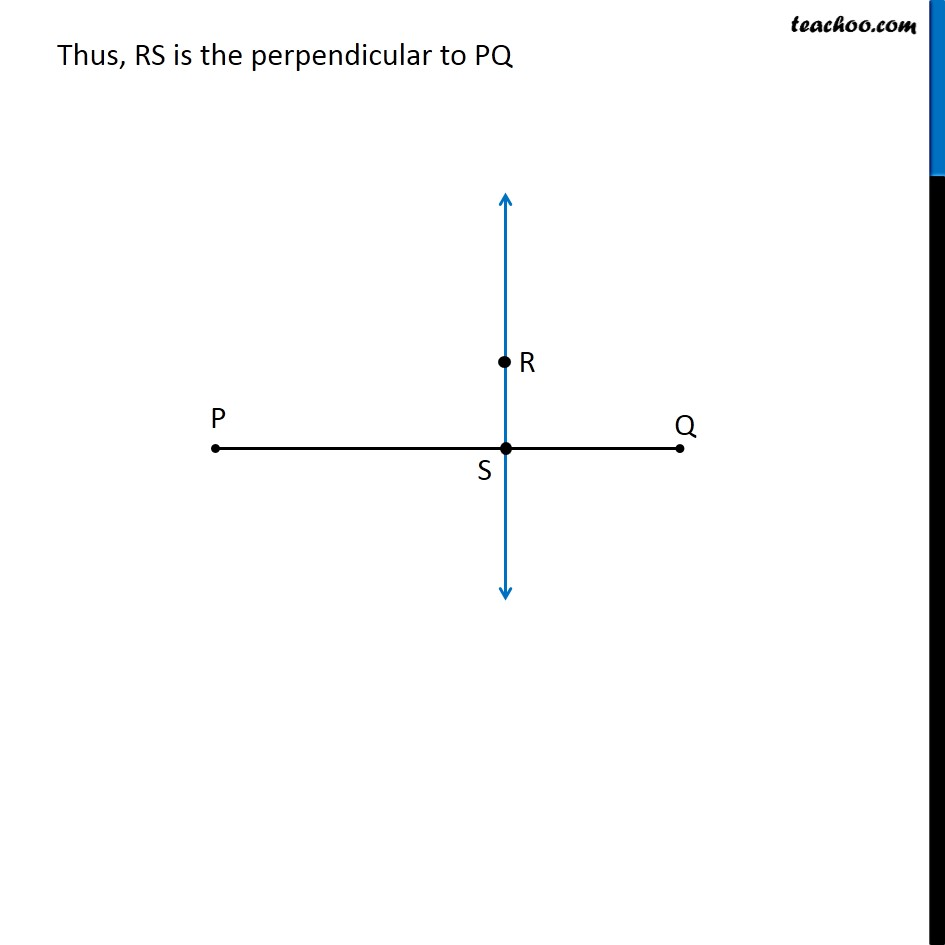Learn in your speed, with individual attention - Teachoo Maths 1-on-1 Class

### Transcript

Question 2 Draw any line segment (𝑃𝑄) ̅ . Take any point R not on it. Through R, draw a perpendicular to (𝑃𝑄) ̅ . (Use ruler and set-square) Let’s take an example 1. Given a line segment PQ with point R not on it Place set square on the line so that line is exactly above the set square 3. Put ruler on the hypotenuse line of the set square 4. Keeping the ruler in the same position, slide the set-square till one end of the set-square passes through point P 4. Keeping the ruler in the same position, slide the set-square till one end of the set-square passes through point P 5. Draw a line along the edge of the set square, keeping set-square firm Let the line intersect line l at S Thus, RS is the perpendicular to PQ